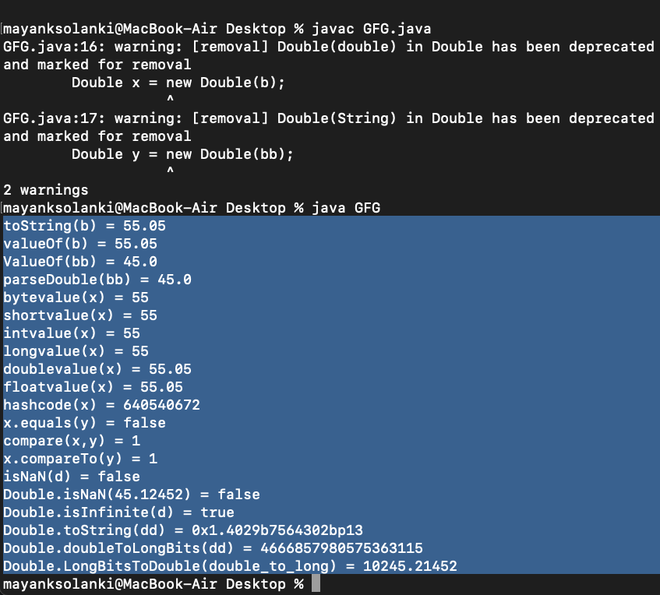Open in App
Not now

# Java.Lang.Double Class in Java

• Difficulty Level : Basic
• Last Updated : 03 May, 2022

Double class is a wrapper class for the primitive type double which contains several methods to effectively deal with a double value like converting it to a string representation, and vice-versa. An object of Double class can hold a single double value. Double class is a wrapper class for the primitive type double which contains several methods to effectively deal with a double value like converting it to a string representation, and vice-versa. An object of Double class can hold a single double value.

There are mainly two constructors to initialize a Double-object.

A. Double(double b): Creates a Double-object initialized with the value provided where it takes a value with which to initialize as a parameter.

`public Double(Double d) `

Parameters: Value with which to initialize

B. Double(String s): Creates a Double-object initialized with the parsed double value provided by string representation where it takes a string representation of the byte value as a parameter.

Default radix is taken to be 10.

`public Double(String s) throws NumberFormatException`

Exception Thrown: It throws NumberFormatException if the string provided does not represent any double value.

## Java

 `// Java Program to Illustrate Double Class``// Via Demonstrating Its Methods`` ` `// Class``public` `class` `GFG {`` ` `    ``// Main driver method``    ``public` `static` `void` `main(String[] args)``    ``{`` ` `        ``// Declaring and initializing``        ``// double and String values``        ``double` `b = ``55.05``;``        ``String bb = ``"45"``;`` ` `        ``// Construct two Double objects``        ``Double x = ``new` `Double(b);``        ``Double y = ``new` `Double(bb);`` ` `        ``// Method - toString()``        ``System.out.println(``"toString(b) = "``                           ``+ Double.toString(b));`` ` `        ``// Method - valueOf()``        ``// Return Double object``        ``Double z = Double.valueOf(b);``        ``System.out.println(``"valueOf(b) = "` `+ z);``        ``z = Double.valueOf(bb);``        ``System.out.println(``"ValueOf(bb) = "` `+ z);`` ` `        ``// Method - parseDouble()``        ``// Return primitive double value``        ``double` `zz = Double.parseDouble(bb);``        ``System.out.println(``"parseDouble(bb) = "` `+ zz);`` ` `        ``// Print statements``        ``System.out.println(``"bytevalue(x) = "``                           ``+ x.byteValue());``        ``System.out.println(``"shortvalue(x) = "``                           ``+ x.shortValue());``        ``System.out.println(``"intvalue(x) = "` `+ x.intValue());``        ``System.out.println(``"longvalue(x) = "``                           ``+ x.longValue());``        ``System.out.println(``"doublevalue(x) = "``                           ``+ x.doubleValue());``        ``System.out.println(``"floatvalue(x) = "``                           ``+ x.floatValue());`` ` `        ``int` `hash = x.hashCode();``        ``System.out.println(``"hashcode(x) = "` `+ hash);`` ` `        ``boolean` `eq = x.equals(y);``        ``System.out.println(``"x.equals(y) = "` `+ eq);`` ` `        ``int` `e = Double.compare(x, y);``        ``System.out.println(``"compare(x,y) = "` `+ e);`` ` `        ``int` `f = x.compareTo(y);``        ``System.out.println(``"x.compareTo(y) = "` `+ f);`` ` `        ``Double d = Double.valueOf(``"1010.54789654123654"``);``        ``System.out.println(``"isNaN(d) = "` `+ d.isNaN());`` ` `        ``System.out.println(``"Double.isNaN(45.12452) = "``                           ``+ Double.isNaN(``45.12452``));`` ` `        ``// Double.POSITIVE_INFINITY stores``        ``// the positive infinite value``        ``d = Double.valueOf(Double.POSITIVE_INFINITY + ``1``);``        ``System.out.println(``            ``"Double.isInfinite(d) = "``            ``+ Double.isInfinite(d.doubleValue()));`` ` `        ``double` `dd = ``10245.21452``;``        ``System.out.println(``"Double.toString(dd) = "``                           ``+ Double.toHexString(dd));`` ` `        ``long` `double_to_long = Double.doubleToLongBits(dd);``        ``System.out.println(``"Double.doubleToLongBits(dd) = "``                           ``+ double_to_long);`` ` `        ``double` `long_to_double``            ``= Double.longBitsToDouble(double_to_long);``        ``System.out.println(``            ``"Double.LongBitsToDouble(double_to_long) = "``            ``+ long_to_double);``    ``}``}`

### Output: